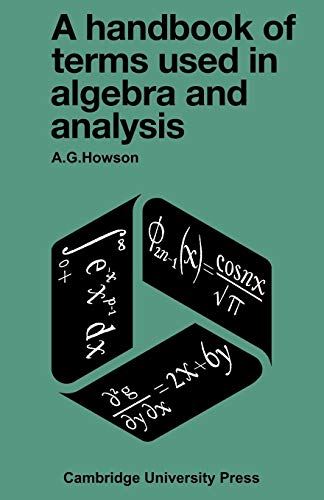# A Handbook of Terms used in Algebra and Analysis by A. G. Howson

In Stock
£6.49
Degree students of mathematics are often daunted by the mass of definitions and theorems with which they must familiarize themselves. In the fields algebra and analysis this burden will now be reduced because in A Handbook of Terms they will find sufficient explanations of the terms and the symbolism that they are likely to come across in their university courses.
Only 2 left

## A Handbook of Terms used in Algebra and Analysis Summary

### A Handbook of Terms used in Algebra and Analysis by A. G. Howson

Degree students of mathematics are often daunted by the mass of definitions and theorems with which they must familiarize themselves. In the fields algebra and analysis this burden will now be reduced because in A Handbook of Terms they will find sufficient explanations of the terms and the symbolism that they are likely to come across in their university courses. Rather than being like an alphabetical dictionary, the order and division of the sections correspond to the way in which mathematics can be developed. This arrangement, together with the numerous notes and examples that are interspersed with the text, will give students some feeling for the underlying mathematics. Many of the terms are explained in several sections of the book, and alternative definitions are given. Theorems, too, are frequently stated at alternative levels of generality. Where possible, attention is drawn to those occasions where various authors ascribe different meanings to the same term. The handbook will be extremely useful to students for revision purposes. It is also an excellent source of reference for professional mathematicians, lecturers and teachers.

### Why buy from World of BooksOur excellent value books literally don't cost the earthFree delivery in the UKEvery used book bought is one saved from landfill

1. Some mathematical language; 2. Sets and functions; 3. Equivalence relations and quotient sets; 4. Number systems I; 5. Groups I; 6. Rings and fields; 7. Homomorphisms and quotient algebra's; 8. Vector spaces and matrices; 9. Linear Equations and rank; 10. Determinants and multi linear mappings; 11. Polynomials; 12. Groups II; 13. Number systems II; 14. Fields and polynomials; 15. Lattices and Boolean algebra; 16. Ordinal numbers; 17. Eigenvectors and eigenvalues; 18. Quadratic forms and inner products; 19. Categories and functors; 20. Metric spaces and continuity; 21. Topological spaces and continuity; 22. Metric Spaces II; 23. The real numbers; 24. Real-valued function of real variable; 25. Differentiable functions of one variable; 26. Functions of several real variables; 27. Integration; 28. Infinite series and products; 29. Improper integrals; 30. Curves and arc length; 31. Functions of a complex variable; 32. Multiple integrals; 33. Logarithmic, exponential and trigonometric functions; 34. Vector algebra; 35. Vector calculus; 36. Line and surface integrals; 37. Measure and lebesgue integration; 38. Fourier series; Appendix 1 some 'named' theorems and properties; Appendix 2 Alphabets used in mathematics; Index of symbols; Subject index.

GOR004719480
A Handbook of Terms used in Algebra and Analysis by A. G. Howson
A. G. Howson
Used - Very Good
Paperback
Cambridge University Press
1972-09-07
252
0521096952
9780521096959
N/A
Book picture is for illustrative purposes only, actual binding, cover or edition may vary.
This is a used book - there is no escaping the fact it has been read by someone else and it will show signs of wear and previous use. Overall we expect it to be in very good condition, but if you are not entirely satisfied please get in touch with us.